## Federated learning for clinical applications

##### A primer for the data-governance/privacy curious, October, 2022### Data Privacy and Governance in Clinical Machine Learning

Clinical machine learning seeks to ethically improve patients’ outcomes in healthcare settings using complex data and statistical methods. Generally, many of these statistical methods involve learning associations between various real-world measures – like the risk of disease X and the amount of protein Y. The accuracy of these learned associations tends to increase as you get more data. Additionally, newly developed methods with the potential to solve unique problems, also tend to require more data to get accurate results. Likewise, models targeting rare conditions require, unsurprisingly, even more data. This is not to say that data is the only issue facing clinical machine learning, but it certainly an important factor in the field's progress. However, the process of collecting storing, and distributing data, especially medical data, is extremely difficult and often not incentivized. We’re going to briefly discuss two important aspects of the data ecosystem -- data privacy, and data governance. We’ll then describe a technical advancement in model training – federated learning – that allows for model development in an ecosystem focused on maintaining decentralized data governance .
Data Privacy: Medical data are often highly sensitive in nature and breaches in privacy could cause significant harm to patients. Additionally, it is becoming clear that simply anonymizing data – i.e. removing patient names and unique identifiers – might not be enough to ensure privacy. We now know that patient faces can be reconstructed from medical scans, making the patient's identifying features and the features used in modeling inseparable in these applications. These issues make hospitals hesitant to share data, even with other hospitals, and put high security requirements on any server seeking to host shared data from multiple sources.
Data Governance: Collecting, storing and maintaining large datasets takes massive amounts of work. In academic settings, providing these datasets as a resource to the public is not incentivized; time spent writing original research papers is considered more useful. Similarly in industry or non-profit settings, institutions often want to retain control of their data efforts because of the resources invested into them. While it would also benefit progress to incentivize sharing, finding ways to work within current incentive structures for data governance can help build larger multisite datasets quickly.
Both data privacy and data governance are necessary parts of the data ecosystem that unfortunately disincentivize institutions to share their data or contribute them to larger data lakes. Making them both an important safeguard and a barrier to progress. If there were a way to decentralize model training such that institutions could retain control of their data, and modelers could incorporate that data into their work, clinical applications could advance without sacrificing privacy or drastically changing incentives. This decentralization is the promise of federated learning.

### Federated Learning Definition

Federated learning achieves decentralization by training individual models at each participating institution and then aggregating the parameters from each local model to create one global model. This way, data are never passed between sites, only parameters are. Before going into a bit more depth on the definition, lets visualize a typical centralized learning pipeline (visualization adapted from ).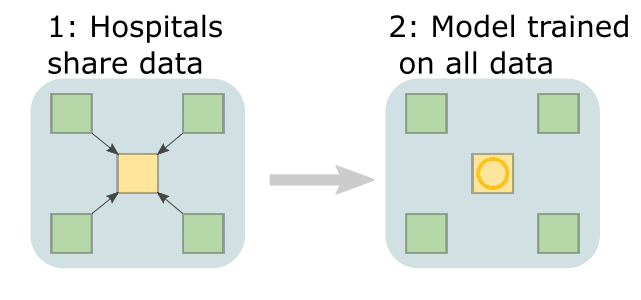Data from multiple hospitals (green squares, called 'nodes') would need to be shared with some central server (yellow square, called 'aggregate node' or 'central node'). Assuming that was successful, you could then train a model on data from every site at that centralized server. Seems simple, but we’ve already discussed why data sharing might not be a sustainable solution. Let instead look at the schematic for federated learning (visualization adapted from ).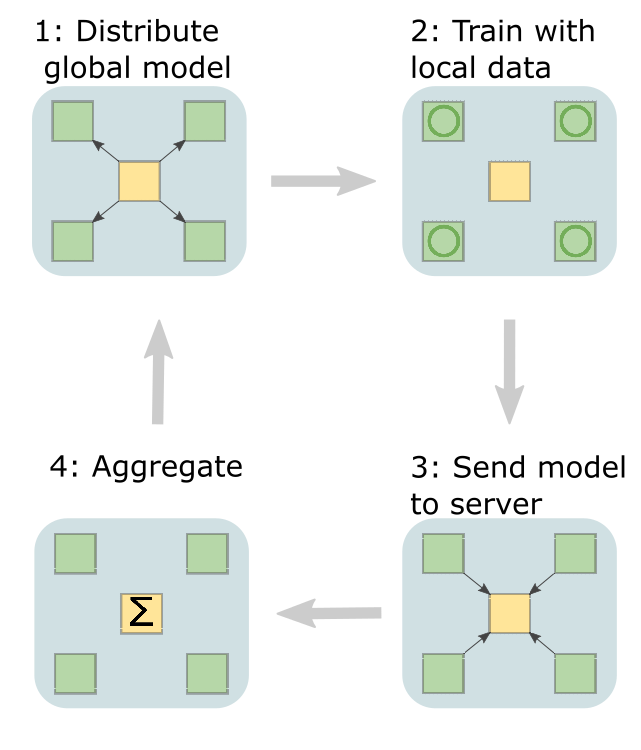The first step is for the central server to share a model with each node. Then, models are trained locally before sending their parameters back to the central server. The central server will then aggregate all the parameters, before sending the updated model back out to each hospital. This repeats until the model is trained. To formalize this definition, let’s think about a standard loss function from a machine learning model. $$\min_{\phi} L(X;\phi)$$ Typically, the goal is to minimize some loss function $$L$$ over different parameters $$\phi$$. Now, we can expand this loss function to accommodate a decentralized framework $$L(X;\phi) = \sum^{K}_{k=1}w_k L_k(X_k;\phi_k)$$ Here $$k$$ indexes each local data source. Now, we are simply minimizing the weighted sum of local losses. Typically, this weighting $$w_k$$ is given by the fraction of observations present at each hospital, but different weighting schemes can serve different purposes (more on this later). It’s important to note that federated learning is huge field, with lots of different flavors and variations. What was described above, and what will be discussed in the remainder of this post is a specific kind that seems natural to many clinical applications. Specifically, these applications involve aggregating data from multiple hospitals, such that each node is a hospital, and each hospital stores mostly the same variables. To facilitate future searches, this method is called centralized federated learning (which is confusing, given that the alternative to federated learning is called centralized learning), using the FedAvg algorithm with a hub and spoke topology.

### Decisions and Considerations when Implementing Federated Learning

Before implementing any federated learning system, there are some precursor decisions you’ll have to make, as well some features of your data that should be quantified. Some of these decisions have standard solutions for the generic medical context (i.e. multiple hospitals, with mostly the same features, but different observations). For the few considerations that don’t have standard solutions, we’ll go over a set of common options in more detail.
Decision or Consideration Description Standard Solution in Clinical Setting
Nodes and Topology How many nodes? Will there be aggregate nodes? How will they be connected? Few nodes (each node is a hospitals), all connected to one aggregate
Updates How many nodes will participate in each update? All connected nodes will participate in updates
Data structure Naming conventions, file structures, etc. None, because it depends so much on the specific problem you’re working with. Here’s an example from neuroscience called BIDS
Data partitions Are features, labels, or observations shared across nodes? Each node will have different observations, but at least some shared features and labels
Data distribution How are features and labels distributed across nodes, and how will this influence the learning algorithm? Multiple (see below)
Privacy measures What extra privacy measures will be taken, if any? Multiple (see below)
Hyper parameters Weighting coefficients (wk), loss function, etc. Weighting by the number of observations, but there are some interesting and useful variants (see dealing with non-IID data for some examples). All other parameters determined similarly to centralized learning
We will discuss the two issues with multiple solutions: data distribution (specifically, non-independent, or non-IID data); and additional privacy.
Dealing with Non-IID Data: The biggest technical issue on this list is probably 'data distributions'. The standard FedAvg algorithm discussed here is not guaranteed to work well when the data are not identically distributed (non-IID) across nodes – and is rarely IID across nodes in real-world settings. Quantifying how the distributions of data differ and adapting the algorithm to deal with those distributions is an important part of the process. Overall, there are three big ways that data can differ across nodes. Nodes can have missing values, nodes can have different distributions or proportions of values, or the same values can lead to different predictions in different contexts. When reviewing the literature, it seems like each of these different data situations has a unique name, however not everyone seems to agree on what that name is. I think you can understand everything in this post without the names, but for the purpose of searching the field, here are the common names I observed for these different data situations:
1. Feature skew: some features not present. i.e. one hospital does not record heart rate.
2. Label distribution skew: some labels are not present. i.e. building a model to predict COVID when one hospital has no patients with COVID.
3. Concept Shift: the same features lead to a different label. i.e. building a model to predict gut health from cheese consumption with hospitals in Asia and Europe. Since lactose intolerance is more common in Asia, the 'cheese' feature would lead to different labels at different hospitals
4. Concept Drift: or the same label arises from different features. i.e. building a model to predict anxiety levels in hospitals with patients from different socioeconomic levels. While both hospitals might have patients with anxiety, the things causing that anxiety might differ.
5. Quantity skew: labels have different distributions (imbalanced). i.e. building a model to predict COVID when one hospital has 40% of patients test positive, and another has 2%.
6. Prior probability shift: features have different distributions. i.e. using age as a predictor when data come from children's hospitals and general hospitals.
7. Unbalancedness: vastly different numbers of observations. This one is self explanatory.
A survey of clinical applications of federated learning before the year 2022 quantified how many papers reported each of the first 5 distributions above. The most common distribution reported was quantity skew, or imbalanced labels across nodes (18/24 papers). Because of its ubiquity, we’re going to go over some common solutions as well.
1. Balancing training data: each node can implement its own resampling scheme, such as SMOTE or GAN resampling. In the same review mentioned above, this was the most popular method for addressing skew , though the review only discussed the first three methods in this list.
2. Adaptive hyperparameters: using loss functions and weighting coefficients that are specific to each node.
• LoAdaBoost: one specific example that boosts the training of weak nodes by forcing the loss function to fall below some threshold before they contribute to the aggregate.
3. Domain adaptation: Use meta-training to determine how to combine predictors trained on various domains, similar to transfer learning.
4. Share data: share a small amount of data or summary statistics from data to fill in missing values and supplement skewed distributions
5. Normalization: (only applied to deep learning models) group, rather than batch normalization helps with skewed labels.
6. Different algorithm: Federated learning based on dynamic regulation (FedDyn) algorithm can guarantee that the node losses converge to the global loss.

Dealing with Privacy: Despite federated learning being more secure than centralized methods, federated learning is not free from privacy risks. Bad actors with access to the model can still reverse engineer data from the model parameters, and therefore gain access to sensitive information. This issue is more pressing when not all the nodes can be trusted – like when nodes are users cell phones rather than hospitals. Because of this, about half of clinical federated learning papers do not use additional privacy protections. However, if you’re interested in adding extra security, there are two ways that people tend to increase security: adding noise, and encryption.
1. Add noise: add noise to either the data, or the gradients
• Differential privacy: a method of adding noise that ensures that model outputs are nearly identical even if any one data point is removed.
2. Encryption: encrypt the gradients or parameters that get sent back and forth

### Python Packages for Implementing Federated Learning

If you’ve thought about the design of your federated learning pipeline and are ready to implement it, there are a few free packages in Python that can help you get your system up and running
1. PySyft
A screenshot from a PySyft tutorial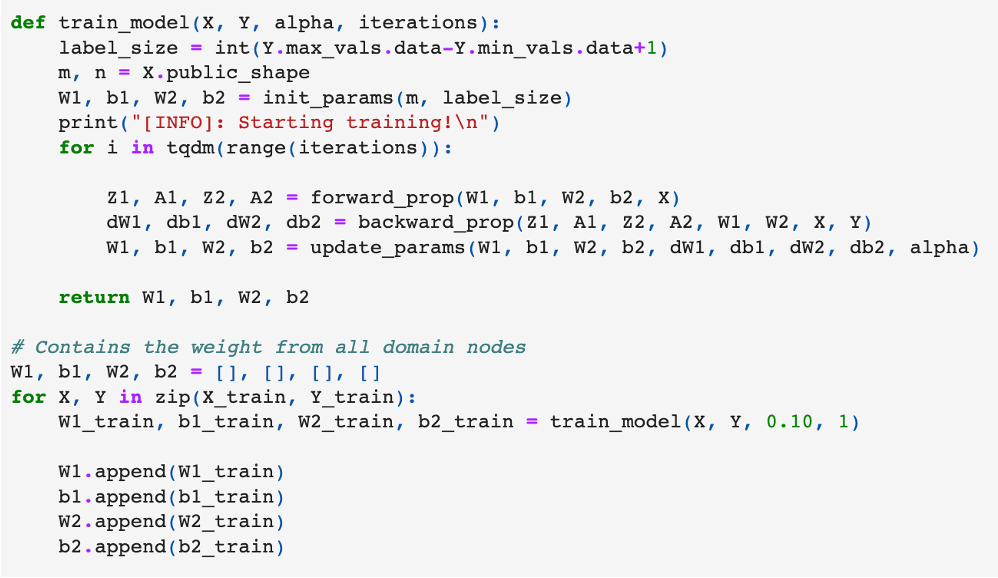• Supports encryption and differential privacy
• Support for non-IID data (via sample sharing)
• ‘numpy-like’ interface (their words)
• Currently, they want users to work with the team on new applications
2. Tensorflow
A screenshot from a tensorflow tutorial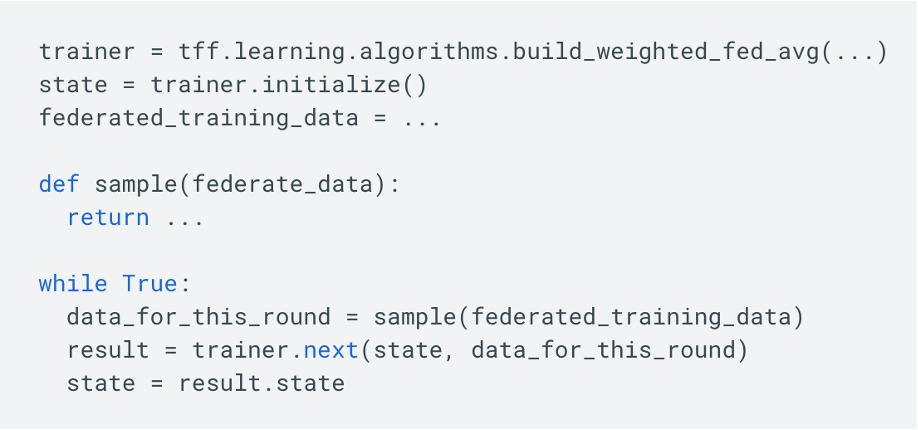• Probably easy to use if you already work with tensorflow
• No built-in support for privacy or non-IID data
3. FATE
A screenshot from a FATE tutorial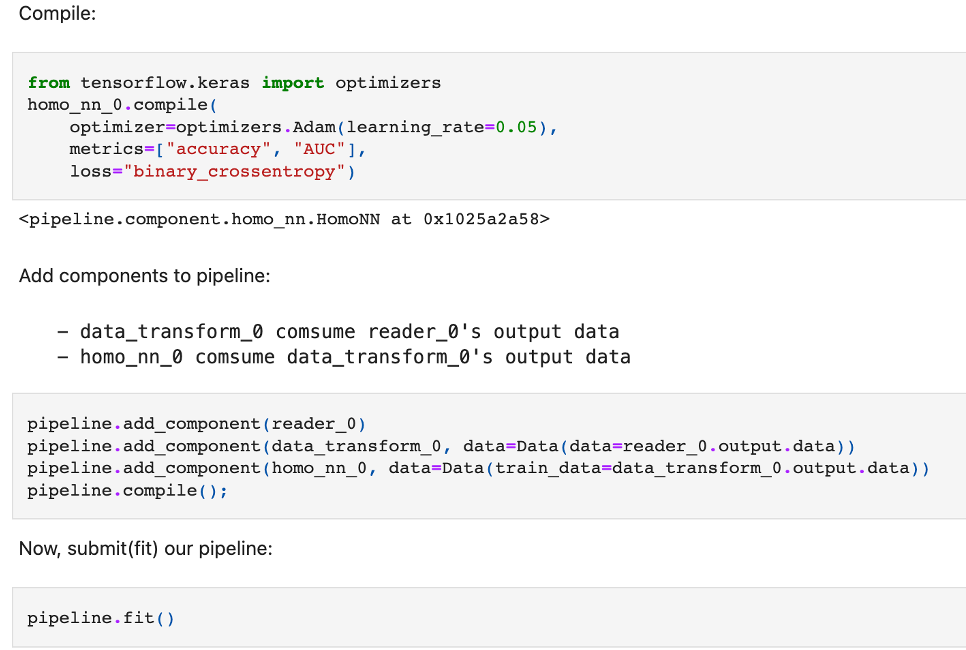• No support for non-IID data (though nothing is stopping you from adding your own resampling function to the pipeline)
• Supports encryption
• Pipeline package interface

### Conclusions and Commentary

• Federated Learning promises a flexible, decentralized way to train machine learning algorithms. Widerspread adoption of federated learning could make modeling with more sophisticated methods, or for more niche populations feasible
• Federated learning is presented as a solution to data governance and privacy issues that make sharing data difficult. While the method has clear benefits over centralized learning, data privacy, and especially data governance, will likely still present issues moving forward. As discussed in the post, federated learning applications are not a complete solution to security issues and will likely require more protections in any real-world application. Additionally, the incentives that make it harder for institutions to contribute data to data lakes might also make it harder to offer access for federated learning projects. If you spent a lot of money collecting a rare dataset, you might want to get the first (or second, or third) crack at any modeling projects using that dataset. Essentially, I do not think federated learning can serve as a substitute for incentivizing data sharing or protecting/compensating data curators.
• Starting a federated learning project requires many decisions and considerations. Decisions with the least clear solutions are those involving data standardization across sites and those involving how to deal with non-IID data distributions. Both reviews cited in this post recognize that these are important issues[1,3], but stop short of providing clear recommendations. I think the method would be more accessible and more likely to be used responsibly if some of the packages produced pandas-profiler style reports of data distributions across sites and provided more support for implementing solutions.

### References and Resources

1. Rieke, N., Hancox, J., Li, W., Milletarì, F., Roth, H. R., Albarqouni, S., Bakas, S., Galtier, M. N., Landman, B. A., Maier-Hein, K., Ourselin, S., Sheller, M., Summers, R. M., Trask, A., Xu, D., Baust, M., & Cardoso, M. J. (2020). The future of digital health with federated learning. Npj Digital Medicine, 3(1).
2. Schwarz, C. G., Kremers, W. K., Therneau, T. M., Sharp, R. R., Gunter, J. L., Vemuri, P., Arani, A., Spychalla, A. J., Kantarci, K., Knopman, D. S., Petersen, R. C., & Jack, C. R. (2019). Identification of Anonymous MRI Research Participants with Face-Recognition Software. New England Journal of Medicine, 381(17), 1684–1686.
3. Prayitno, Shyu, C. R., Putra, K. T., Chen, H. C., Tsai, Y. Y., Tozammel Hossain, K. S. M., Jiang, W., & Shae, Z. Y. (2021). A systematic review of federated learning in the healthcare area: From the perspective of data properties and applications. In Applied Sciences (Switzerland) (Vol. 11, Issue 23). MDPI
4. Huang, L.; Yin, Y.; Fu, Z.; Zhang, S.; Deng, H.; Liu, D. LoAdaBoost: Loss-based AdaBoost federated machine learning with reduced computational complexity on IID and non-IID intensive care data. PLoS ONE 2020, 15, e0230706.
5. Guo, J., Shah, D. J., & Barzilay, R. (2018). Multi-Source Domain Adaptation with Mixture of Experts
6. Hsieh, Kevin; Phanishayee, Amar; Mutlu, Onur; Gibbons, Phillip (2020-11-21). The Non-IID Data Quagmire of Decentralized Machine Learning". International Conference on Machine Learning. PMLR: 4387–4398.
7. Acar, Durmus Alp Emre; Zhao, Yue; Navarro, Ramon Matas; Mattina, Matthew; Whatmough, Paul N.; Saligrama, Venkatesh (2021). Federated Learning Based on Dynamic Regulation
8. For a lighter read, check out this comic from Google

## Coastal differences in artists' Instgram captions

##### A network analysis of tattoo pieces, September, 2022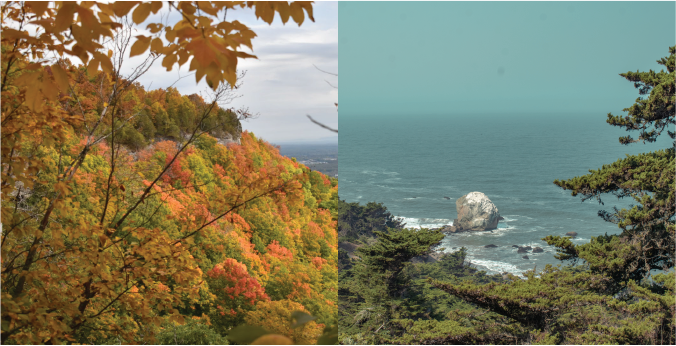To me, tattoos seem like a great way to express autonomy, aesthetics, and interests all in one place. I'm always interested in hearing peoples tattoo stories, and my Instagram feed is usually about 70% images of fresh ink on any given day. Recently I've wondered what sorts of stories I might be missing by limiting my exposure to hyper-curated algorithms and people I already know. In short, I wanted to do a broader survey of the tattoo landscape. If I could pull enough data and do some statistical clustering, I could potentially sort through a much larger swath of the tattoos than I would normaly be exposed to. Specifically, I wanted to answer a few questions: What are the most common tattoo styles and subjects? How do these differ geographically in the US? If I can get descriptors of each tattoo, what are some prominent groupings of tattoo descriptors? Do those groupings differ in different cities? And, are there any interesting groups I haven't been exposed to so far? I decided to use Instagram posts to collate a dataset of tattoos, and to use hashtags from each post as markers of style, content, or other tattoo features. As a simple assessment of geographic specificity, I pull two datasets: one from San Francisco (SF) and one from New York (NY). I use these datasets to create a network representation of tattoos in each city. The network representation stores relations between posts and hashtags and allows me to identify clusters of similar posts and their content.

### Common tags in SF and NY

From Instagram, we can get the top 10,000 posts from a specific hashtag (i.e. #sftattooartists, or #nyctattooartists). Each post has some information associated with it - the number of likes, the account that posted it, the hashtags, etc. Here we're going to throw out all this information except the hashtags. We now have bunch of posts, and all the tags used to describe them. Below, you can see the number of posts associated with the most popular hashtags. San Francisco tags are in cool colors, and New York tags are in warm colors.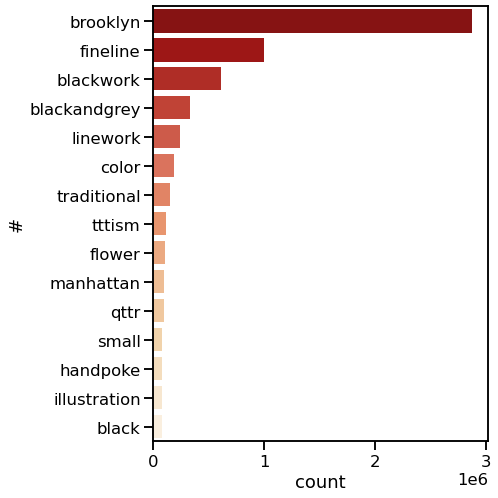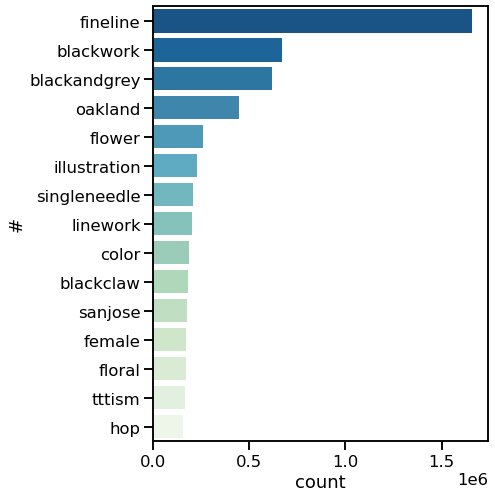A lot of the popular tags break down into a few categories.
• Artist location: Artists include hashtags for nearby locations such as #brooklyn or #sanjose. These tags were probably originally something like #brooklyntattoo or #sanjosetattoo artist that got clipped in the hashtag cleaning process. It is also possible artists are making tattoos of these actual regions, though I think this is less likely.
• Tattoo subject: In both cities, flowers are a popular subject for tattoos (#floral, #flower), though this is the only subject the cracks the top 10.
• Tattoo style: A few styles like #fineline, #blackwork, #color and #blackandgrey a top tags in both cities. A few styles that are considered popular but don’t make the cut in both would be #traditional, #newschool, #japanese, etc.
• Tattoo magazines:
• One tag, #tttism, is a tattoo magazine with a large digital division
One notable omission to me was hashtags about tattoo locations. While there are clearly more popular tattoo placements (#wristtatoo vs #facetattoo), no placements end up in the top 10. This is likely because many posts include a mixture of finished pieces and artwork that could theoretically end up anywhere. What about the biggest differences between cities? Below, we see the difference in the percentage of posts containing each tag, for each city.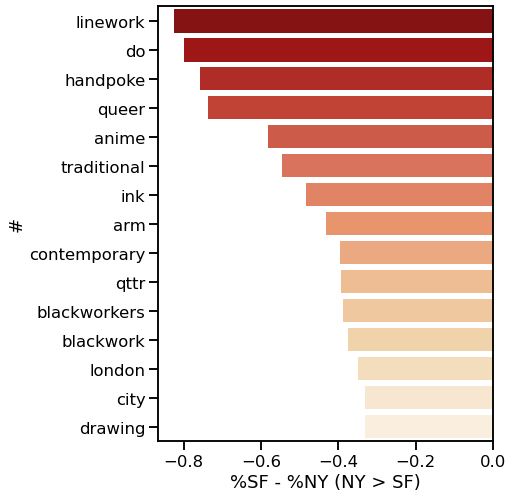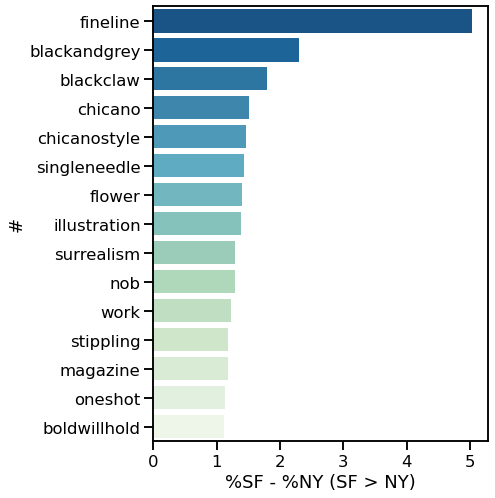These separate into different categories.
• Artist identity: New York has a higher prevalence of both #queer and #qttr (which is an abbreviation for queer tattoo artist). Does this mean that there are more queer artists in New York? There are a few possible explanations. It is possible that there are more queer artists in NY, that queer artists in NY are more willing to self identify on social media, or that queer SF artists use different or more specific terminology.
• Tattoo techniques: One surprising difference to me was the prevalence of tags referencing different tattoo equipment on different coasts. Specifically, #handpoked tattoos, which are non-electric, are more popular in NY, while #singleneedle tattoos, which use only one kind of needle, are more popular in SF
• Tattoo style: One of the most interesting differences to me is the dichotomy between #traditional and #surrealist style tattoos on each coast. Surrealism constitutes a more niche style than traditional tattoos in general, and its prevalence in SF evokes images of the cities free-spirited, pre-silicon valley past for me. Similarly, #chicano and #chicanostyle are not often in listed in the US's top styles, but their growing popularity in a state with growing Latinx population seems intuitive.
• Tattoo subject: We find that NY has a much higher prevalence of anime tattoos compared to SF. However, it is once again possible that SF tattoo artists simply use different, or more specific language to tag their anime tattoos.
We've learned a lot about the coastal tattoo landscape just from looking at popular hashtags. But there is higher level information that this technique ignores. Which tags tend to be grouped together? What subjects, styles, and placements? To answers these questions about groups of descriptors, we are going to move on to our network analysis.

### Instagram tattoo posts as networks

We can represent all the Instagram posts and their hashtags in a network like the one below. Nodes in this network are both posts (shown on the left), and tags (shown on the right). Lines connect posts to their corresponding tags.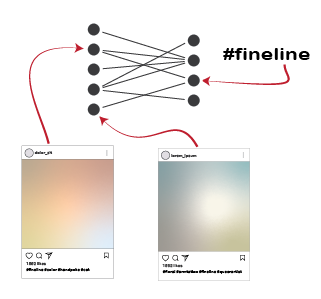If you want to know how to make a network like this using Python, you can see the code here. To find groups in graphs, we are going to use a method that assigns distances to items based on how similar their connectivity is. So, two posts with the same hashtags would have a small distance, and posts with very different hashtags would have a large distance. We can then group the posts and hashtags such that distances are small within a group, and big between groups. But how many groups do we pick? If the best grouping gives us 10,000 groups is that useful? Rather than picking a specific number of groups, we're going to use a nested approach. This means that we're going to start with big groups, then look for smaller groups within those big groups, and repeat this process until our groups are very small. We will end up with a gradient of grouping descriptions that range from coarse-grained (few, large groups) to fine-grained (many, small groups). This way we can pick the resolution that suits our current questions best. Specifically, we're going to use a method called the nested weighted stochastic block model, that makes very few assumptions about the structure of groups and works especially well with big data. Below, we can see the network visualization of our two tattoo post networks. The left hand side shows posts, and the right hand side shows hashtags. The colors correspond to the different groups (at the most fine-grained level). You can also see the hierarchical structure of the nested groups overlayed on top in light blue.

### Network clusters of tattoo posts

New York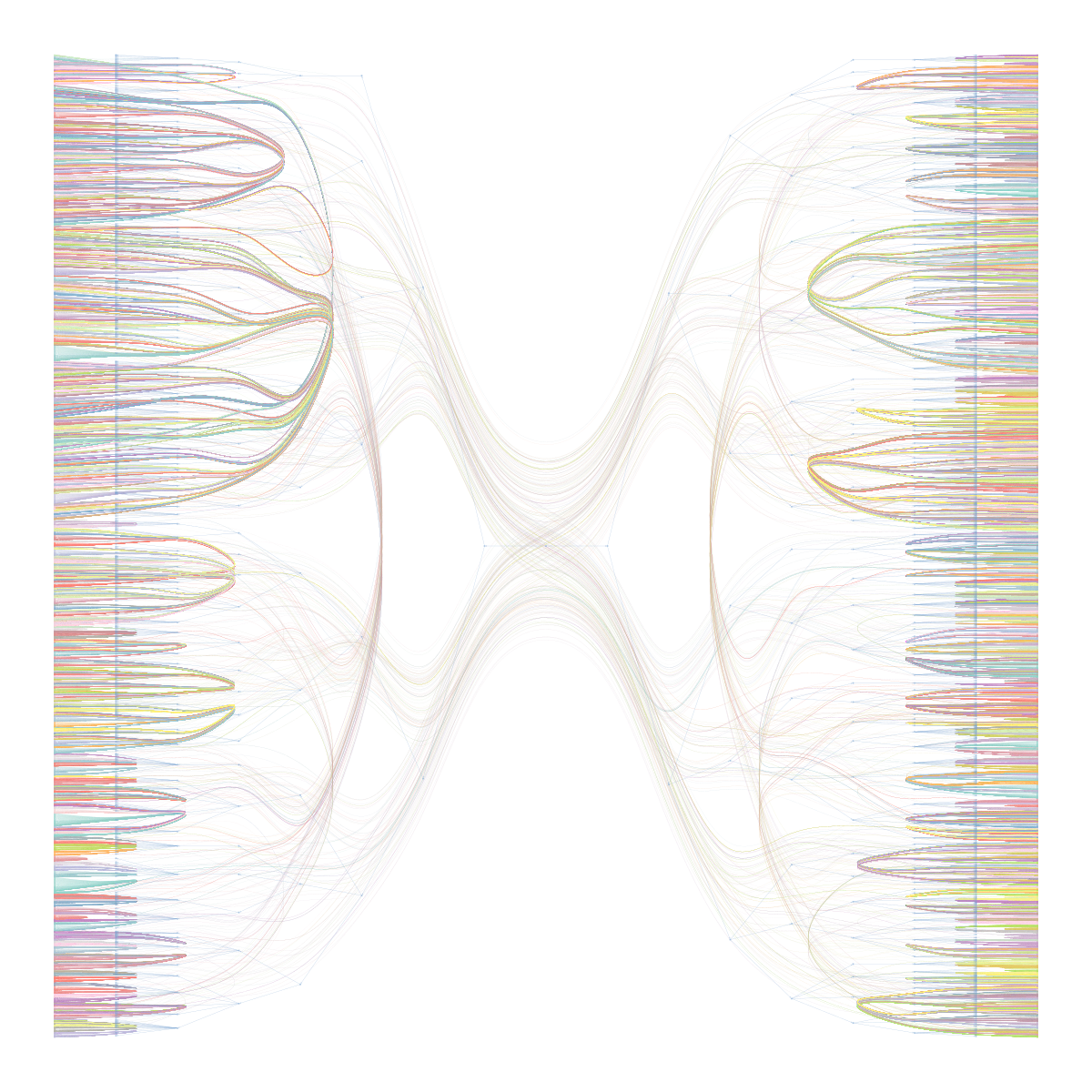San Fransisco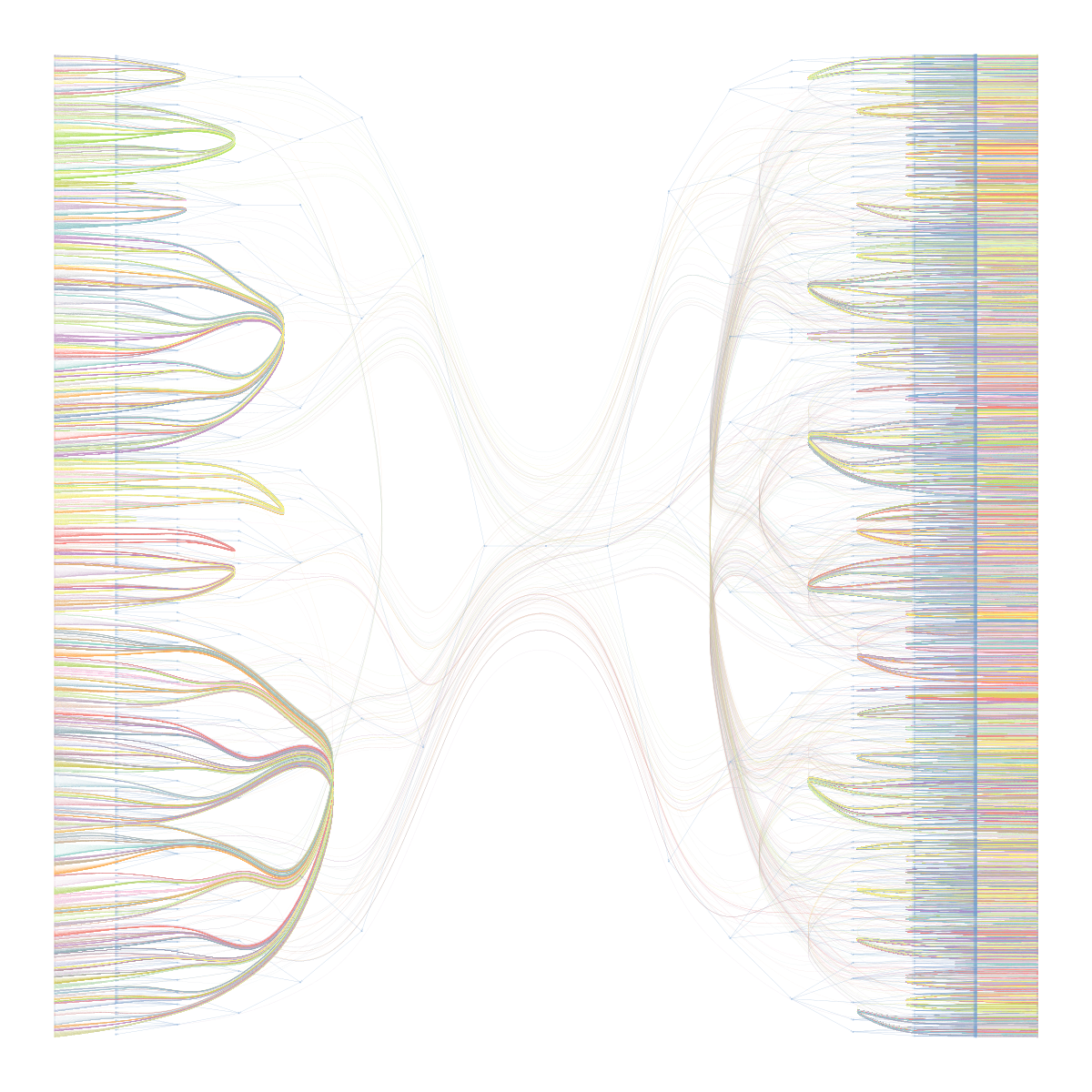There are some prominent differences between the grouping structure of the two cities.
Nested CLusters in New York
1. Level 1: 2 groups
2. Level 2: 3 groups
3. Level 3: 7 groups
4. Level 4: 20 groups
5. Level 5: 52 groups
6. Level 6: 160 groups
7. Level 7: 637 groups
8. Level 8: 28282 groups
Nested Clusters in San Fransisco
1. Level 1: 2 groups
2. Level 2: 5 groups
3. Level 3: 14 groups
4. Level 4: 32 groups
5. Level 5: 98 groups
6. Level 6: 263 groups
7. Level 7: 1463 groups
New York has more levels than San Francisco, though the number of groups per level grows faster in San Francisco. Additionally, we find that the grouping in NY is 'better', meaning that within/between group distance separation is larger. This suggests a slightly less fragmented Instagram tattoo post landscape in New York, where smaller numbers of groups give the best distance separations. We can now pick a level with relatively few groups (Level 4) and visualize the different hashtags in each group. If hashtags are in the same group, that means that there is some overlap in the posts containing those tags. In the plots below, we can make word clouds to represent each group in level 4. The size of the word indicates the number of posts in the group that have the hashtag, and the size of the word cloud indicates the total number of posts in the group. The color indicates nothing, it's just for readability.
Hashtags in New York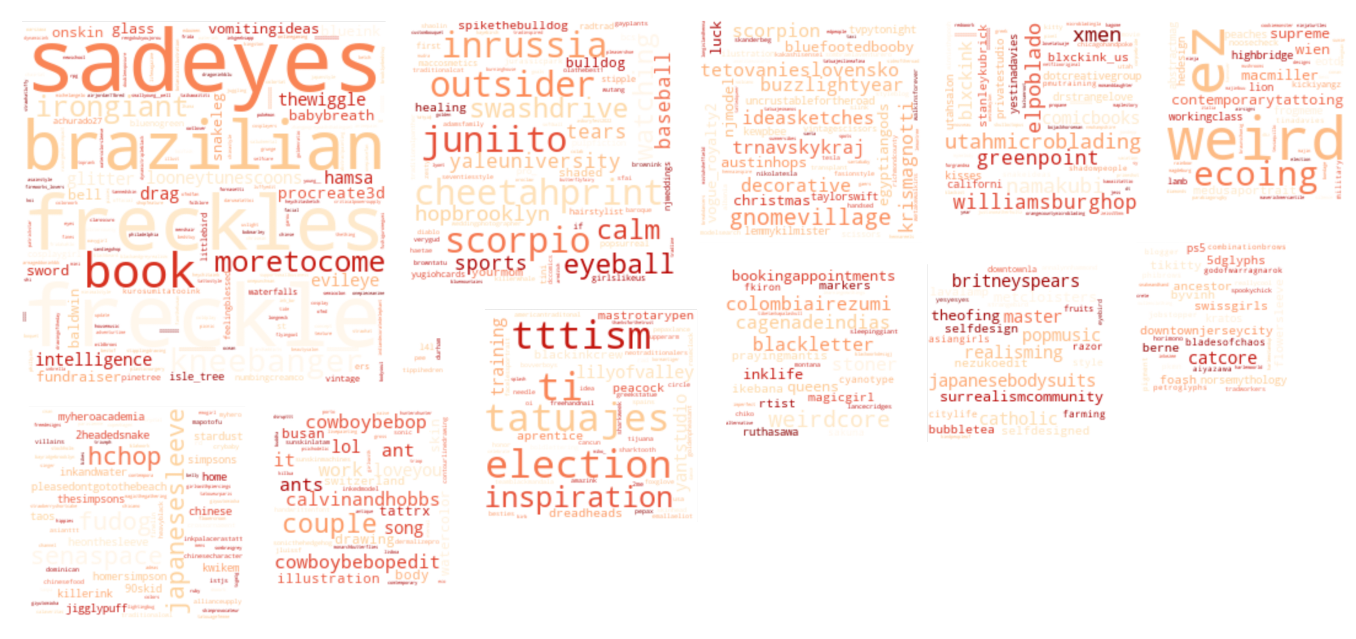Hashtags in San Fransisco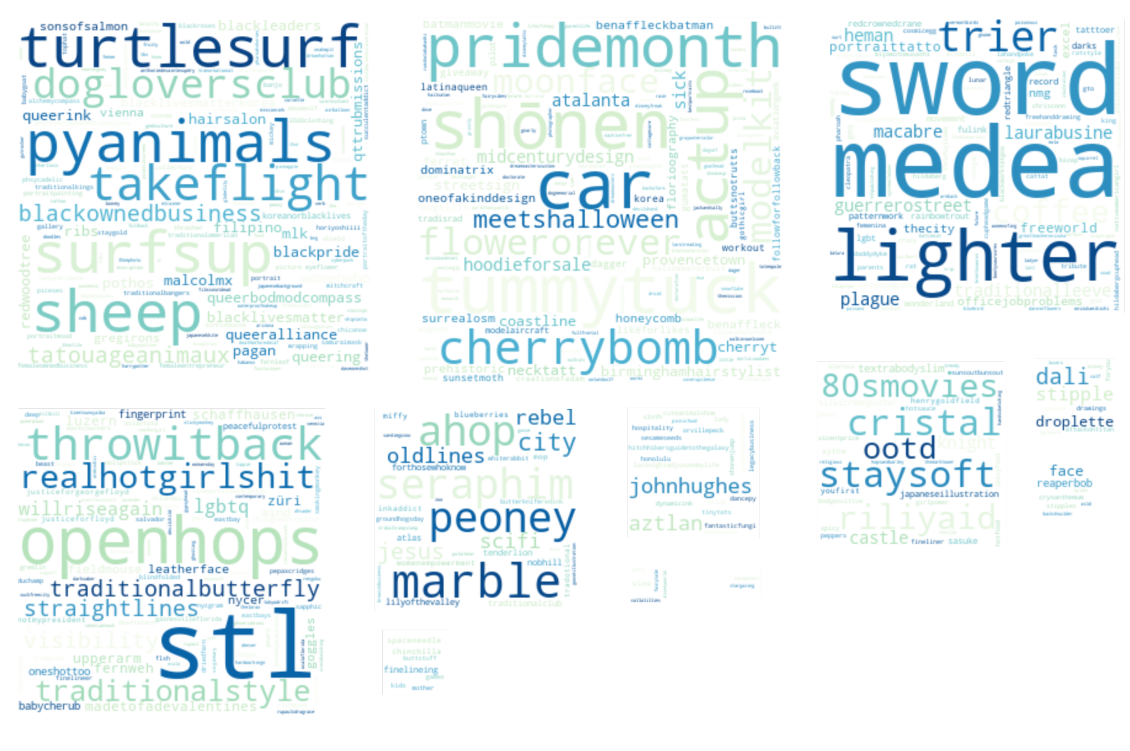We can also see some interesting differences in this visualization. New York has communities that are more evenly sized, while San Francisco has are more small groups. Lastly, we can look at a finer scale and visualize the hashtags contained in some of the smaller communities.
We get some communities that are expected:
Cats and Pets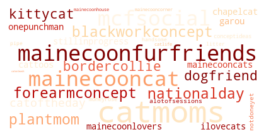Black and grey animals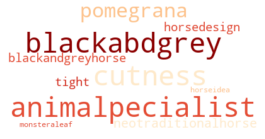Flowers and Skulls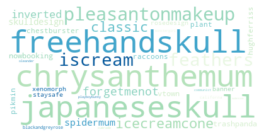Pokemon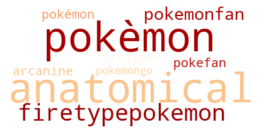Neotraditional tattoos with white inkSpace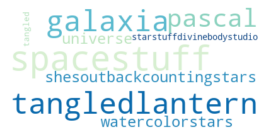Others that are less expected, but show consistent themes:
AgricultureGhost type pokemon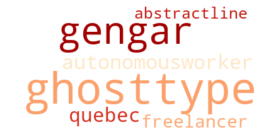Animal prints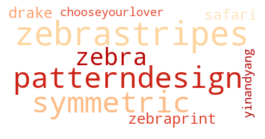Cartoon portraits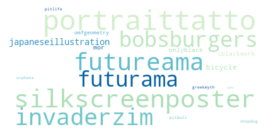Chickens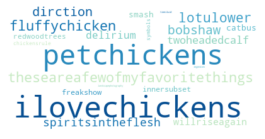Tattoo artists giving themselves ocean themed leg tattoos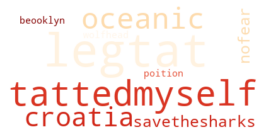And lastly, some that show some confusing and inspiring mixes of topics:
Psychedelic cheese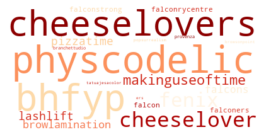Batman (the Ben Affleck one) and plants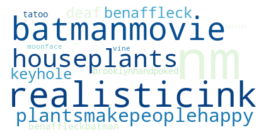Indi(ana Jones) music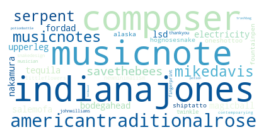#### Conclusions

• We find that regardless of city, fine line, black work, and black and grey styles are popular to tag. Similarly, flowers or floral designs are consistently mentioned
• San Francisco has a uniquely large community of artists posting about Chicano style and surrealist tattoos, while New York has more posts about anime tattoos and traditional styles
• We find some evidence that New York tattoo posts more easily separate into large groups, while San Francisco tattoos have more small, niche groups.
• A nonzero number of people have tattoos of the Ben Affleck batman
I do think I'm leaving this project with a better understanding of the tattoo landscape, at least as it is represented on Instagram. I've also learned how to work with a rich representation of transient cultural attitudes and am looking forward to finding some other fun applications of the method.

## How many roads must a random walker walk down before it gets out of Reykjavik?

##### Analyzing your Google location history and biased random walkers on street graphs (in Python), August, 2022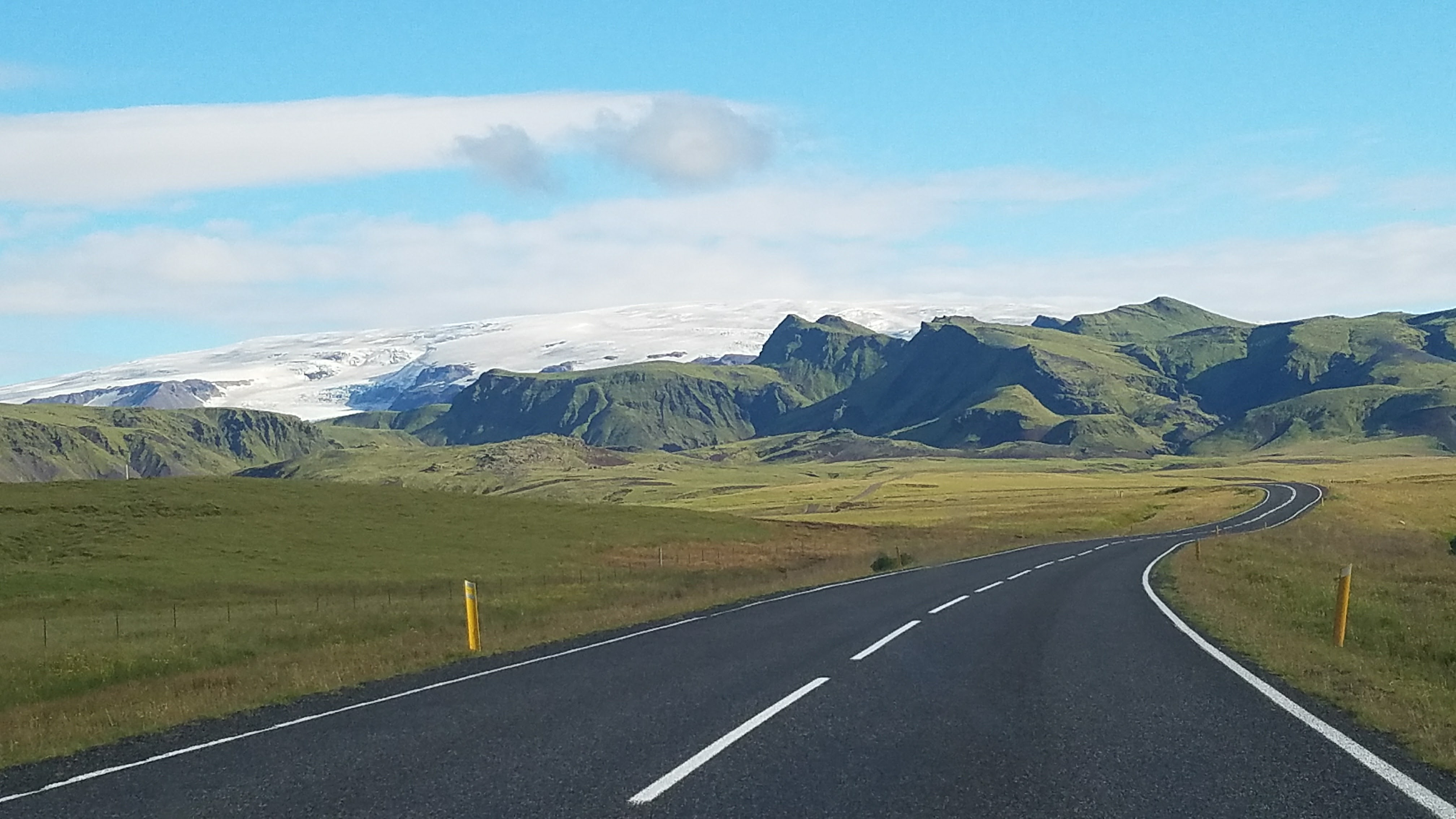I spent a good part of my PhD learning about how people explore spaces. I specifically was interested in abstract spaces, where distances in these spaces map on to the similarity between items. These items could be emotions, objects, school subjects, birds, or anything else you want. It turns out lots of the theories for how how humans learn and explore abstract spaces also hold water when examining how we or other animals explore physical spaces. These cross domain theories felt very satisfying to me; it seemed like they might at best be revealing something important about our behavior, and at worst showing cool convergent behavioral motifs. I wanted to take some of my knowledge of exploration, as well penchant for learning new data vis methods, to learn something about my own exploration style through physical space. What sorts of cost functions might I have in mind when traveling? How well have I really explored my city? How much are my paths driven by novelty, or familiarity? This blog post will answer none of these questions. But it will go through the steps I took to learn a few things that are fundamental to answering them: the structure of Google maps location data; and how to analyze road networks and implement random walks in Python. Along the way, we'll answer a much simpler and more pointless question: does my vacation to Iceland bear any qualitative similarity to a biased random walker?

### Google location data for exploration

I'm going to use my own Google location data to visualize my path through Iceland. I followed these steps to download the data. The data come in two sets, a more information rich folder called Semantic Location History, and sparser json file called Records. We're working with only the Records data here. The important features of this data are latitudes and longitudes, as well as time stamps. Coordinates will be mapped to publically available road networks using the package OSMnx, and timestamps will be used to order and filter data. We can get rid of all the extra columns and clean up the ones we want like so:

import pandas as pd
import json

with open('YOUR_PATH_TO_DATA/Takeout/Location History/Records.json') as data_file:
df = pd.json_normalize(data, 'locations')

# get only relevant variables
df = df[['latitudeE7', 'longitudeE7', 'timestamp']]

# tranform them to be useful
df.timestamp = pd.to_datetime(df.timestamp)
df = df.assign(
lat=df['latitudeE7']/1e7,
long=df['longitudeE7']/1e7,
year=df['timestamp'].dt.year,
month=df['timestamp'].dt.month,
day=df['timestamp'].dt.day,
dow=df['timestamp'].dt.day_name(),
time=df['timestamp'].dt.time,
)


As a quick validity check, let's use the geopandas package to visualize all our different coordinates. Google has data on my locations since 2012, so this plot should include most of my locations over the past decade (i.e. concentrated on the two US coasts).

import geopandas as gpd
from shapely.geometry import Point
import matplotlib.pyplot as plt

# set up data structure of coordinates
geo = [Point(xy) for xy in zip(df.long, df.lat)]
gdf = gpd.GeoDataFrame(df, geometry=geo)

# plot
gdf.plot(ax=world.plot(figsize=(14,14), color='lightgrey'), color="#7397CB")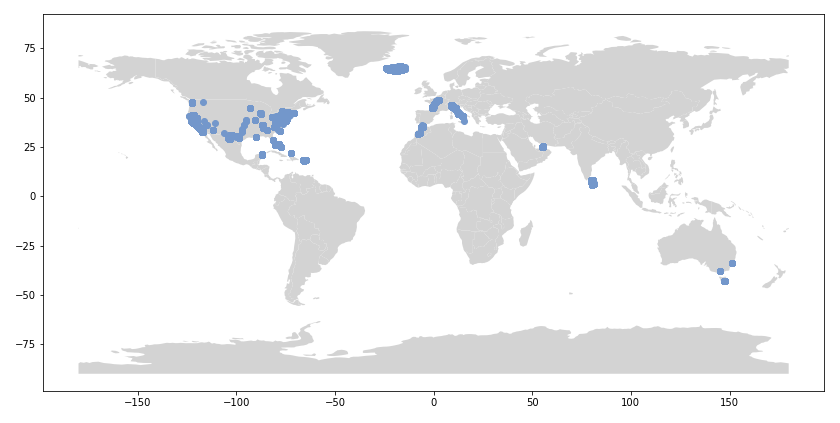Great! Now we're going filter down to the specific week where I was in Iceland

st =  '2017-07-31'
en = '2017-08-07'
iceland = df[(df['timestamp'] >= st) & (df['timestamp'] <= en)]

# remove duplicate lat/long pairs
iceland = iceland.drop_duplicates(subset=['lat', 'long', 'day', 'month'])

Now that we have the data we'll be working with, we're ready to move on to working with road networks.

### Visualizing your paths through road networks

We'll be using two Python packages to help visualize my path through Iceland: OSMnx, and networkX. OSMnx has data about road maps for most of the world. networkX allows us to use graph data structures to get information about the road networks. Both packages leverage some ideas and terminology from graph theory that we're going to go over before starting. A network or graph is a representation of system of two or more separable units (called nodes) and the interactions between them (called edges). Visually, nodes are often depicted as circles, with edges between them depicted as lines. In our case, edges are going to be roads and nodes are any intersections or decision points in those roads.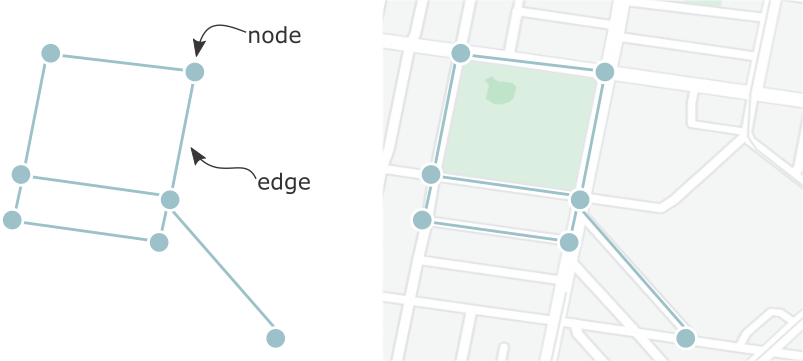First, let's get a graph representation of Iceland's roads

import osmnx as ox

ox.config(log_console=True, use_cache=True)
# location of graph
place     = 'Iceland'
# find shortest route based on the mode of travel (for our purposes it doesnt really matter which one)
mode      = 'walk'        # 'drive', 'bike', 'walk'
# find shortest path based on which feature
optimizer = 'length'        # 'length','time'
# create graph
graph = ox.graph_from_place(place, network_type = mode)

What exactly is this graph object we just made?

type(graph)


Output:

            networkx.classes.multidigraph.MultiDiGraph

This is a networkX object. This means we can use functions and attributes from networkX to get properties of the graph. For example, we can get the density (or proportion of possible connections present) and number of edges in this graph.

import networkx as nx

print(nx.density(graph))
print(len(graph.get_edges()))


Output:

            1.9575729910546824e-05

            327816

We're now going to use networkX to get paths between nodes that correspond to my location data. Our strategy for calculating my trajectory on this graph is as follows: get a starting coordinate; snap it to the closest spot on the road network; get an ending coordinate; snap it to the network; get the shorted path between the start and end coordinate; repeat. We can code up this strategy as follows:

route = []
start_latlng = (iceland['lat'].values,iceland['long'].values)

# find the nearest node to the start location
orig_node = ox.get_nearest_node(graph, start_latlng)

# get the rest of the path
for i in range(len(iceland) - 1):
# define the end location in latlng
end_latlng = (iceland['lat'].values[i+1],iceland['long'].values[i+1])
# find the nearest node to the end location
dest_node = ox.get_nearest_node(graph, end_latlng)
#  find the shortest path
route.extend(nx.shortest_path(graph,
orig_node,
dest_node,
weight=optimizer))
# advance to the next step
orig_node = dest_node

And then plot it

from itertools import groupby

# remove any duplicates from the route - these cause the plotting to break
route = [i for i in groupby(route)]
fig, ax = ox.plot_graph_route(graph,
route,
node_size=0,
edge_linewidth=0.3,
edge_color='white',
route_color="#EB8D43",
route_linewidth=1.5,
route_alpha=1.0,
orig_dest_size=0)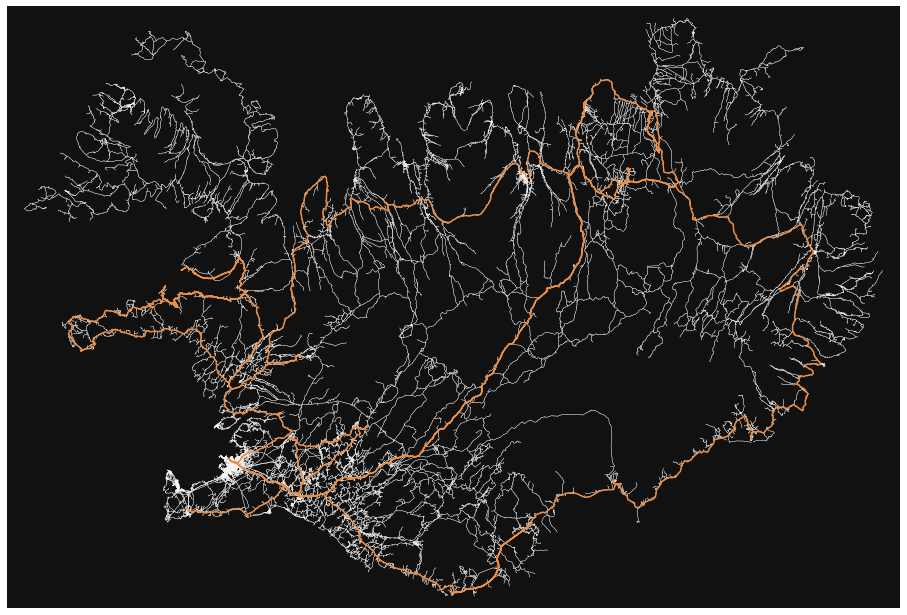And here we have a visualization of my vacation. It's also important to note some limitations in this visualization. This method requires snapping to a road network. This might get incorrect locations at times when I was not on a true road, like on hikes or boats. Additionally, the shortest path between two points might not be the path that I took. This can lead to wrong data especially in cases where I travelled far between Google's recordings. Given these caveats, we can still observe some things about my path. While I did travel through most parts of the country, the path I took is very directed. No one area has dense coverage, and the path mostly sticks to the coast. These observations make sense given what I wanted to get out of the trip. I wanted to see as much of the country as possible in the week I was there. I also had prebooked accomodations in different cities for every night, which didn't allow me to wander or dwell in any one area. How could this have looked different? What if I had showed up to Iceland and just wandered the streeets of Reykjavik, turning randomly whenever I felt like it? What if I turned mostly randomly, but had tried to avoid places I had been before?

### Random walkers

All these strategies can be coded up by imagining some agent who stands on a given node, and iteratively 'walks' to other connected nodes based on some rules. The rules are called 'biases', and sometimes even simple biases can lead to agents with behaviors that closely mimic real-world behaviors. Even when they don't, they can identify how much of the variance in a behavior is explained by simple rules and help better identify which features we don't yet understand. We'll go over a few examples below We'll start off looking what an unbiased random walker would do one the streets of Iceland (starting in Reykjavik), for a few different path lengths

# scaling factor for length of walk
c = 1 # plots shown for 1, 2, and 10
# intialize
start_latlng = (iceland['lat'].values[i],iceland['long'].values[i])
orig_node = ox.get_nearest_node(graph, start_latlng)
random_route = [orig_node]

# get the walk
for k in range(n):
# pick a node of the proper step size
next_node = list(graph.neighbors(orig_node))
# get random selection for next step
dest_node = np.random.choice(next_node)

# only add to our route
random_route.append(dest_node)
orig_node = dest_node

$$c=1$$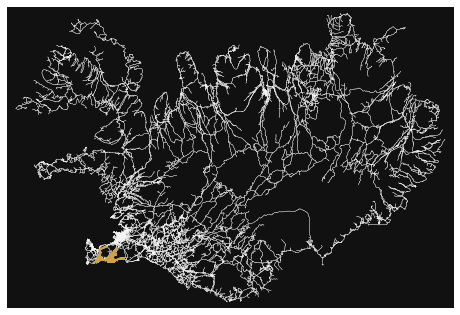$$c=2$$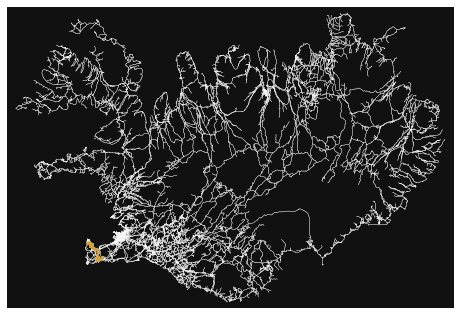$$c=10$$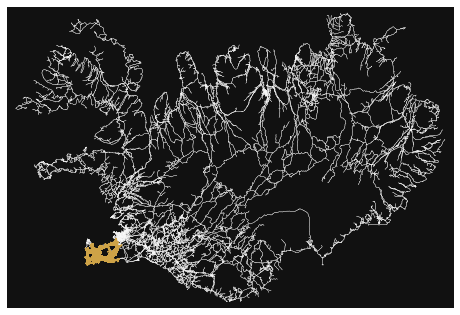This algorithm looks a lot different from my path! It doesn't see the whole country, and in fact never really gets far out of Reyykjavik, even if it takes 10 times more steps than I do. This isn't surprising given how much more densely connected streets are inside of cities than outside of them. Once you're inside a city, more intersections will lead you into the city than out of it, leading to a spot where random walkers will tend to accumulate. Maybe if we try to to avoid intersections we've already seen, we can make sure that we get out of the city eventually.

We can add biases to this walk algorithm to make it less likely to revisit nodes that its already been too. This is accomplished by adding an additional parameter ($$r$$) that sets the relative probability of revisiting nodes already in the path versus visiting new ones.

r = 0.01

# get the walk
for k in range(n):
# get neighboring nodes
next_node = list(graph.neighbors(orig_node))
# get transition probabilities, weighted by revisits
transition_prob = np.ones((len(next_node),))
revisit_idx = [m in bias_random_route for m in next_node]

# check if all nodes are revisits/new
if (len(set(revisit_idx)) == 1):
# set equal probability
transition_prob = transition_prob*(1/len(transition_prob))
else:
# parameter r determines bias
transition_prob[revisit_idx] = r*transition_prob
# normalize so it sums to 1
transition_prob = transition_prob/sum(transition_prob)

# get random selection for next step
dest_node = np.random.choice(next_node, size=1, replace=False, p=transition_prob)
dest_node = dest_node # unwrap from list

# only add to our route
bias_random_route.append(dest_node)
orig_node = dest_node

$$c=1$$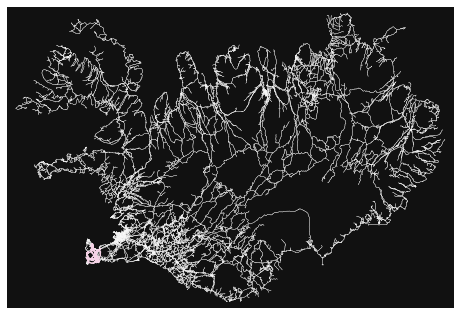$$c=2$$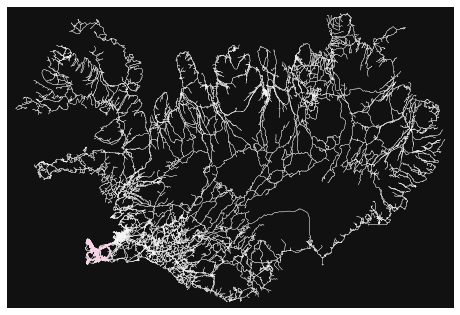$$c=10$$This bias towards novelty does a little better. Now, we much more easily get out of the city, and even get to a different part of the island. But the areas it visits are still more densely explored than mine, and I still get more coverage of the island as whole.

There's a lot of ways we could build on this bias towards novelty to get more realistic looking walks. We could add a bias towards popular locations, force the walk to start and stop at the airport, or give the walker global knowledge of the graph and tell it to navigate efficiently to specific landmarks. But for now, we'll stop here, and appreciate the aesthetic differences in these few exploration styles.

## Double/debiased machine learning

##### Light- to medium-math explanation of the method with tutorials, June, 2021### What is double machine learning

To me -- and potentially, its creator -- Double Machine Learning sounds like a trendy name you would give a method to try to sound impressive in developer spaces. Double (or debiased) machine learning is actually a way to estimate specific causal effects in large, complex data . Previously many causal modeling methods relied on assuming a specific form of the data rather than learning it - namely assuming that variables were normally distributed and linearly related to each other. In addition to these faulty assumptions, these methods don't allow for complex data where the entropy of the parameter space increases with increasing observations (in other words - most large, modern datasets). Combined, these features made causal inference difficult to apply to realworld problems. A method called double machine learning (DML) allows causal inference to coexist with complex data with few assumptions, which has drummed up a lot of well-deserved excitement about the method (primarily in economics). When I was trying to learn more about DML, I found that there weren't as many resources out there as I had hoped and that most of the resources that were out there took a very theoretical approach. I wanted to create a resource that explained the theory at a higher level and had a larger emphasis on code based explainations. This post focuses on understanding how the DML algorthim works. I hope later to do a post on its application, but for now I'll point people to this tutorial.

#### What clinical problems can DML be used for?

The creation of this methods aligns nicely with some trends in clinical informatics (my current field). Clinical machine learning projects have made a major push towards building risk or diagnostic models. Less attention has been devoted to using machine learning to suggest treatments or interventions. DML presents one path forward - for a particular causal structure. We can illustrate that structure like this: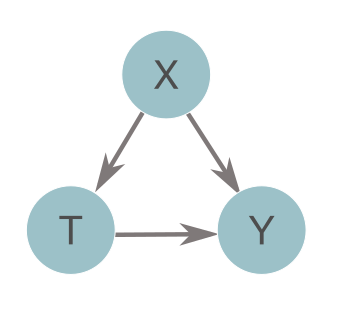Here, Y is some clinical outcome of interest (risk of disease, probability of diagnosis, etc.). T is some treatment or intervention. X is all the relevant demographic, medical and social features of the patients. This diagram is illustrating that the treatment will influence the risk of disease, and that features of the patient will influence both the risk and which/whether treatments will be given. When clinician are deciding which treatments to give, it would be helpful to know the size of the arrow connecting T and Y (referred to now as the treatment effect). Specifically, we'd want the estimation of the treatment effect to:
1. be accurate with a lot of data (might seem obvious, but this is harder than it sounds)
2. come with confidence intervals
3. not make strict assumptions about the form of the data (leverage machine learning)
A method that provides these kinds of treatment effect estimates could prove to be a powerful tool for clinical bioinformatics moving forward.

#### DML's solution

These points have historically been hard to acheive because methods for 'good' causal estimates typically do not give us point 3, and methods of machine learning typically do not give us point 1 (and sometimes 2). Machine learning models do not give good causal estimates for 2 reasons:
1. Regularization, necessary for fitting complex data, induces a bias (think bias variance trade-ff). To reduce overfitting, analysts using machine learning methods often use regularization. However, this necessarily increases the bias of estimates.
2. Despite our best efforts, machine learning models fit on data that follow the causal diagram above tend to overfit data, further biasing results.
DML can remove those two sources of bias and give us an estimate of the treatment effect and all the extra points outlined above. At a high level, these biases are alleviated by fitting two separate machine learning models (thus the name) to estimate the effect of X on Y and T, and then solving for theta using the residuals of those estimates (more details on this below). Additionally, there are now some pretty good packages implementing DML in python that play nicely with scikit learn. All together, making it a desireable new method for applied scientists, and motivating me to give it a try.

#### Caveats and alternatives

Like all methods, DML comes with important assumptions and caveats. Assumptions (most of these are true of many causal methods):
1. Consistency - An individual's potential outcome under their observed exposure history is precisely their observed outcome.
2. Positivity - Everyone in the study has some probability of receiving treatment
3. You are recording all variables that influence Y and T in X. I think this is the most fraught assumption in medical contexts .
Caveats:
1. categorical treatment - at the moment, there isn't a way to use DML for a categorical treatment variable that also provides confidence intervals. Other methods, such as doubly robust learning, might be better suited here.
2. biased data classes - DML is known to be biased in cases where one outcome is extremely rare (though it is less biased than many other methods). Over/undersampling of the data might be helpful in these cases.
Alternatives

### How DML allows causal inference and machine learning to mix

We're now going to describe the method in more detail than the above summary. The goal here is to hit the major points of the DML paper  restructured for a more applied audience.

#### Direct method

To formalize the problems and solutions discussed above, we're going to have to be more mathematically precise with our definitions. We're going to start by defining a specific formula for generating data. $${Y = T\theta_{0} + g_{0}(X) + U, E[U | X,T] = 0]}$$ $${T = m_{0}(X) + V, E[V | X] = 0}$$ Let's walk through the terms:
• $$X$$ the features
• $$Y$$ the outcome
• $$T$$ the treatment (it can be binary, continuous, or categorical)
• $$g_0(x)$$ some mappong of x to y, excluding the effect of T and $\theta_0$
• $$m_0(x)$$ some mapping of x to t
• $$\theta$$ - the treatment effect. Here its a scalar, for simplicity, but this doesn't have to be the case
• $$U, V$$ the noise, which cancels out on average
This equation is essentially formalizing the graph we had displayed earlier.These equations are a useful example because they give us a specific functional form for how $$T$$ affects $$Y$$ ($$T \times \theta_0$$). Since this relationship is linear, it makes some of the math a little bit nicer. In the end, we want DML to work for more than just this specific situation, but this definition is useful for now. If we were to code up these relationships in python, it would look something like this. Note that to code this up we must pick a specific $$g_0(X)$$ and $$m_0(X)$$. It could be whatever you want, but here we're using some exponential sums of the first few columns of X (I picked this because that's what the original paper does).

from scipy.linalg import toeplitz
# pick any value for theta_0
theta = -0.4

# define a function for generating data
def get_data(n, n_x, theta):
"""
partially linear data generating process

Inputs:
n       the number of observations to simulate
n_x     the number of columns of X to simulate
theta   a scalar value for theta
"""
cov_mat = toeplitz([np.power(0.7, k) for k in range(n_x)])
x = np.random.multivariate_normal(np.zeros(n_x), cov_mat, size=[n, ])

u = np.random.standard_normal(size=[n, ])
v = np.random.standard_normal(size=[n, ])
m0 = x[:, 0] + np.divide(np.exp(x[:, 2]), 1 + np.exp(x[:, 2]))
t = m0 + u
g0 =  np.divide(np.exp(x[:, 0]), 1 + np.exp(x[:, 0])) + x[:, 2]
y = theta * t + g0 + v

return x, y, t
>
Let's imagine you're given some X, T, and Y data, as well as the data generating equations above. You're then asked to estimate what theta is. One first attempt might be to build one machine learning model of $$T\theta_{0} + g_{0}(X)$$ and $$g_0(X)$$, then regress out $$T$$ to solve for $$\theta_0$$. This is a little tricky because $$g_0(X)$$ is not the influence of $$X$$ on $$Y$$, its the influence of $$X$$ on the part of $$Y$$ that isn’t influenced by $$T \times \theta_0$$. Therefore, we have to do this iteratively: get an initial guess for $$\theta_0$$ in order to estimate $$g_0(X)$$; then use that estimate of $$g_0(X)$$ to solve for $$\theta_0$$. In code, the direct method would look like this: First, we'd simulate our data, and build our machine learning estimate of $$Y$$ from $$T\theta_{0} + g_{0}(X)$$ (we'll call this model $$l_0(X)$$)

from sklearn.ensemble import RandomForestRegressor

# get data
x, y, t = get_data(n, n_x, theta)

# this will be our model for predicting Y from X
ml_l = RandomForestRegressor()
ml_l.fit(x,y)
>
Note that you could use whatever machine model you want, it doesn't have to be a random forest. In this example, it should be anything that can estimate exponential functions (since that's the form we picked for our data generating function). Next, we can take an initial guess for $$\theta_0$$, and then fit our estimate of $$g_0(X)$$

# this will be our model for predicting Y - T*theta from X, or g0_hat
ml_g = RandomForestRegressor()

# initial guess for theta
l_hat = ml_l.predict(x)
psi_a = -np.multiply(t, t)
psi_b = np.multiply(t, y - l_hat)
theta_init = -np.mean(psi_b) / np.mean(psi_a)

# get estimate for g0
ml_g.fit(x, y - t*theta_init)
g_hat = ml_g.predict(x)
>
Lastly, we can regress the effect of $$T$$ our from our prediction

# compute residuals
u_hat = y - g_hat
psi_a = -np.multiply(t, t)
psi_b = np.multiply(t, u_hat)

# get estimate of theta and and SE
theta_hat = -np.mean(psi_b) / np.mean(psi_a)
psi = psi_a * theta_hat + psi_b
err = theta_hat - theta
J = np.mean(psi_a)
sigma2_hat = 1 / len(y) * np.mean(np.power(psi, 2)) / np.power(J, 2)
err = err/np.sqrt(sigma2_hat)
>
If we repeat this process 200 times, we can genereate a histogram of our error term and see how well we did.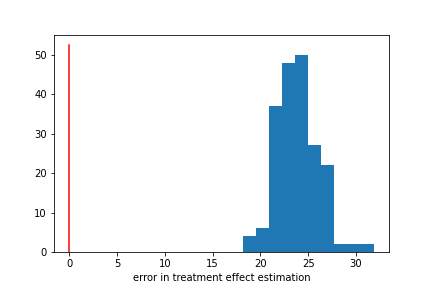If our estimate is good, we would expect the normalized difference between our estimate of $$\theta$$ and the real theta to be centered on 0. This histogram shows that is not the case. Our estimate is way off and centered on a positive value. What went wrong? At a high level, part of what went wrong is that we did not explicitly model the effect of $$X$$ on $$T$$. That influence is biasing our estimate. Illustrating this explicitly is where our partially linear data generating process comes in handy. We can write out an equation for the error in our estimate. The goal here would be for the left-hand side to converge to 0 as we get more data.

#### regularization bias

$$\sqrt{n}(\hat{\theta_0} - \theta_0) =$$ $$(\frac{1}{n}\sum_{i\in I}^nT_{i}^2)^{-1}\frac{1}{\sqrt{n}}\sum_{i \in I}^nT_{i}U_{i}$$ $$+$$ $$(\frac{1}{n}\sum_{i\in I}^nT_{i}^2)^{-1}\frac{1}{\sqrt{n}}\sum_{i \in I}^nT_{i}(g_0(X_i) - \hat{g_0}(X_i))$$
• The left hand side is our scaled error term - what we want to go to 0
• Noise cancels out on average, so this term is a very small number, divided by a big number. Essentially 0
• This term is where the problem is. Our estimate error is never going to be 0. This because of the deal we make as data scientists working with complex data. Reduce the varaince (overfitting) of our machine learning model, we induce some bias in our estimate (often through regularization). Additionally, $$T$$ depends on $$X$$, and therefore also will not converge to 0. Because of this, $$g_0-\hat{g} \times T$$ will be small, but not 0. It will be divided by a large number, and will converge to 0 eventually, but too slowly to be practical.
We have to remove the effect of $$X$$ on $$T$$ to circumvent this bias. We can do this in three steps:
1. Estimate $$T$$ from $$X$$ using ML model of choice (different from the direct method!)
2. Estimate $$Y$$ from $$X$$ using ML model of choice
3. Regress the residuals of each model onto eachother to get $$\theta_0$$
We can write out a new error equation like so: $$\sqrt{n}(\hat{\theta_0} - \theta_0) =$$$$(EV^2)^{-1}\frac{1}{\sqrt{n}}\sum_{i \in I}^nU_iV_i$$ $$+$$ $$(EV^2)^{-1}\frac{1}{\sqrt{n}}\sum_{i \in I}^n(\hat{m_0}(X_i) - m_0(X_i))(\hat{g_0}(X_i) - g_0(X_i))$$ $$+ ...$$
• The left hand side is the same as before
• Noise cancels out on average, so this term is a very small number, divided by a big number. Essentially 0
• Now we have two small, non-0 numbers multiplied by eachother, divided by a large number. This will converge to 0 much more quickly than before
• ... this method adds a new term that we're going to ignore for now. But it comes back later!
In code, this new process only differs in the estimation $$g_0(X)$$ amd $$\theta_0$$. So fitting $$l_0(X)$$ will be the same, but then we have:

# model for predicting T from X - new to the regularized version!
ml_m = RandomForestRegressor()
# model for predicting Y - T|X*theta from X
ml_g = RandomForestRegressor()

ml_m.fit(x,t)
m_hat = ml_m.predict(x)

# this is the part that's different
v_hat = t - m_hat
psi_a = -np.multiply(v_hat, v_hat)
psi_b = np.multiply(v_hat, y - l_hat)
theta_init = -np.mean(psi_b) / np.mean(psi_a)

# get estimate for G
ml_g.fit(x, y - t*theta_init)
g_hat = ml_g.predict(x)
>
Similarly, when we get our final estmimate for $$\theta$$

# compute residuals
u_hat = y - g_hat
# v_hat is the residuals from our m0 model
psi_a = -np.multiply(v_hat, v_hat)
psi_b = np.multiply(v_hat, u_hat)

theta_hat = -np.mean(psi_b) / np.mean(psi_a)
psi = psi_a * theta_hat + psi_b
err = theta_hat - theta
J = np.mean(psi_a)
sigma2_hat = 1 / len(y) * np.mean(np.power(psi, 2)) / np.power(J, 2)
err = err/np.sqrt(sigma2_hat)
>
If we plot a similar histogram over 200 simulations, we'll get something like this: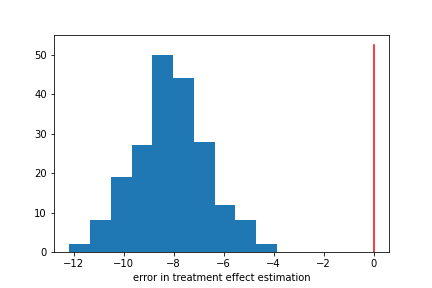And we have greatly reduced (but not eliminated) our bias! For this specific data generating process, we now have a way of estimating $$\theta$$ without regularization bias! However, I mentioned earlier that we want to be able to estimate more than only this process. In particular, step three involves linear regression, and only works in our partially linear example. How do we generalize the method of estimating $$\theta$$? The least squares solution for linear relationships essentially finds the parameters for a line that minimizes the error between the predicted points on the line, and the observed data. We can write this as the minimization of a cost function of our data and true parameters $$\psi(W; \theta, \eta) = 0$$ This equation looks vary different but contains a lot of the same players as before:
1. $$W$$ is the data ($$X$$,$$Y$$, and $$T$$)
2. $$\theta$$ is the true treatment effect
3. $$\psi$$ is just some cost function. We are purposely not defining it because we want this to be a general solution, but you can think of it as any kind of error minimization function
4. $$\eta$$ is called the nuisance parameter, and here contains $$g$$ and $$m$$
Solving minimization problems like these are often difficult and subject to noise. To assure we find a robust solution, we're going to add one other condition to our equation (called a moment condition) $${ \delta_{\eta}E[\psi(W; \theta, \eta][\eta - \eta_0] = 0}$$ Technically, this is a direction al Gateaux derivative. Practically, what this means is that we expect that the true value of $$\theta$$ would be robust to small purturbations in the nuisance parameters. This has the benefit of giving use estimates that will be stable in the presence of small changes to our ML models. There are whole branches of mathematics dedicated to solving these types of equations with moment conditions, and there is no single good solution. All the different solutions are called 'DML estimators'. Rather than getting into any specific estimator here, we're just going to trust that they exist, and move on. Whatever package you use to apply the method should give some information on the estimators it implements. We now have a more generalizable set of steps
1. Estimate $$T$$ from $$X$$
2. Estimate $$Y$$ from $$X$$
3. Solve moment equation to get $$\theta$$

#### overfitting bias

We now have a generalizable solution to regularization bias. Additionally, with the definition of a cost function, we have a method of evaluating our DML estimator and comparing different models. Specifically, we can find the model that gives smallest value for our moment condition. The specific value of this function is usually called a 'score' or 'Neyman orthogonality score', and the closer to 0 it is the better. We would use this value to perform model selection when applying this method. Now, it's time to revisit out error equation in the partially linear case. $${ \sqrt{n}(\hat{\theta_0} - \theta_0) = (EV^2)^{-1}\frac{1}{\sqrt{n}}\sum_{i \in I}^nU_iV_i + (EV^2)^{-1}\frac{1}{\sqrt{n}}\sum_{i \in I}^n(\hat{m_0}(X_i) - m_0(X_i))(\hat{g_0}(X_i) - g_0(X_i)) + \frac{1}{\sqrt{n}}\sum_{i \in I}^{n}V_i(\hat{g_0}(X_i) - g_0(X_i)) }$$ We've discussed the first two terms already, but I'm revealing the last term we had hidden previously. If any overfitting is present in $$\hat{g_0}$$, the estimate will pick up some noise from the noise term $$U$$. This will slow down the convergence of this new term to 0. The solution to this bias is to fit $$g$$ and $$m$$ on a different set of data than the set used to estimate $$\theta$$. Like how cross-validation avoids overfitting during parameter selection, this method (called cross-fitting) avoids overfitting in our estimation of $$\theta$$. This changes our DML steps slightly.
1. Split the data into $$K$$ folds. For each fold:
2. Estimate $$T$$ from $$X$$ using ML model of choice and fold $$K$$
3. Estimate $$Y$$ from $$X$$ using ML model of choice and fold $$K$$
4. Solve moment equation get $$\theta$$ using other sets of data
5. Select $$\theta$$ estimate that gives the best solution over all splits.
In code, all this does is add a loop over folds:

from sklearn.model_selection import KFold
# number of splits for cross fitting
nSplit = 2
x, y, t = get_data(n, n_x, theta)

# cross fit
kf = KFold(n_splits=nSplit)
# save theta hats, and some variables for getting variance in theta_hat
theta_hats = []
sigmas = []
for train_index, test_index in kf.split(x):
x_train, x_test = x[train_index], x[test_index]
y_train, y_test = y[train_index], y[test_index]
t_train, t_test = t[train_index], t[test_index]

ml_l = RandomForestRegressor()
ml_m = RandomForestRegressor()
ml_g = RandomForestRegressor()

ml_l.fit(x_train,y_train)
ml_m.fit(x_train,t_train)
l_hat = ml_l.predict(x_test)
m_hat = ml_m.predict(x_test)

# initial guess for theta
u_hat = y_test - l_hat
v_hat = t_test - m_hat
psi_a = -np.multiply(v_hat, v_hat)
psi_b = np.multiply(v_hat, u_hat)
theta_init = -np.mean(psi_b) / np.mean(psi_a)

# get estimate for G
ml_g.fit(x_train, y_train - t_train*theta_init)
g_hat = ml_g.predict(x_test)

# compute residuals
u_hat = y_test - g_hat

psi_a = -np.multiply(v_hat, v_hat)
psi_b = np.multiply(v_hat, u_hat)

theta_hat = -np.mean(psi_b) / np.mean(psi_a)
theta_hats.append(theta_hat)
psi = psi_a * theta_hat + psi_b
sigma2_hat = 1 / len(y_test) * np.mean(np.power(psi, 2)) / np.power(J, 2)
sigmas.append(sigma2_hat)
err = np.mean(theta_hat) - theta
err = err/np.sqrt(np.mean(sigmas))

Using this process, we can correct the bias in our estimation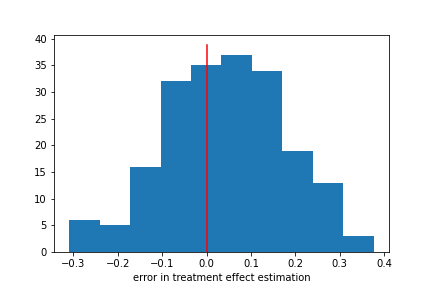Now we have a pretty good estimate! So far, we've gone over what the DML method is, and how is overcomes biases from regularization and overfitting to get good estimates of $$\theta$$ without making strong assumptions about the form of the data. There are two packages (econML and DoubleML) that allow for applications of this method in Python and R. Hopefully this post made the method little more accessible or helped you assess if this method would be a good fit for your data.

### References

1. Chernozhukov, V., Chetverikov, D., Demirer, M., Duflo, E., Hansen, C., Newey, W., & Robins, J. (2016). Double/Debiased Machine Learning for Treatment and Causal Parameters. http://arxiv.org/abs/1608.00060
2. Rose, S., & Rizopoulos, D. (2020). Machine learning for causal inference in Biostatistics. In Biostatistics (Oxford, England) (Vol. 21, Issue 2, pp. 336–338). NLM (Medline). https://doi.org/10.1093/biostatistics/kxz045